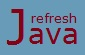# Java Bitwise and Bit Shift Operators with Examples

As the name itself suggests, Bitwise and Bit shift operators operates on bits(`0` and `1`). They operates on binary representation of operands. These operators can be used with integer(`byte, short, int` and `long`) data type variables or values only and they always returns a numerical value. Let's see these operator types one by one.

## Bitwise operators in Java

As the name itself suggests, bitwise operator performs operation bit by bit wise. Table below shows the list of all bitwise operators in java. Consider `a` and `b` as two integer type variables.

Operator Name Example Description
& Bitwise AND Operator a & b Performs bitwise AND operation between bits of `a` and `b`
| Bitwise OR Operator a | b Performs bitwise OR operation between bits of `a` and `b`
^ Bitwise XOR Operator a ^ b Performs bitwise exclusive OR operation between bits of `a` and `b`
~ Bitwise complement Operator ~ a Inverts the bits of variable `a`

All the bitwise operators except complement operator are binary operators. The bitwise complement operator is a unary operator, as it requires only one operand to perform the operation. It belongs to bitwise operator type because it works on bits.

### Bitwise AND operator in Java

Bitwise AND(`&`) operator performs bitwise AND operation on corresponding bits of both the operands. It returns `1` if both the bit's are `1` else it returns `0`. For example & operation between two `byte` variable with value as `53(00110101)` and `79(01001111)` will result in `5(00000101)`.

``` 1 & 1 = 1
1 & 0 = 0
0 & 1 = 0
0 & 0 = 0
```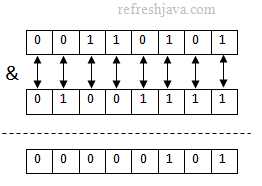### What is the difference between & and && operator in java ?

The & operator performs bitwise AND operation on bit representation of both operands and returns an integer value while logical AND(&&) operator performs operation on two boolean expressions and returns boolean value(`true` or `false`) as result.

### Bitwise OR operator in Java

Bitwise OR(`|`) operator performs bitwise OR operation on corresponding bits of both the operands. It returns `1` if any of the bit's is `1` else it returns `0`. For example | operation between two `byte` variable with value as `53(00110101)` and `79(01001111)` will result in `127(01111111)`.

``` 1 | 1 = 1
1 | 0 = 1
0 | 1 = 1
0 | 0 = 0
```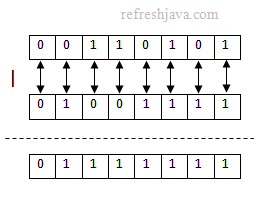### What is the difference between bitwise OR(|) and logical OR(||) operator in java ?

The | operator performs bitwise OR operation on bit representation of both operands and returns an integer value while logical OR(||) operator performs operation on two boolean expressions and returns boolean value(`true` or `false`) as result.

### Bitwise Exclusive OR operator in Java

Bitwise XOR(`^`) operator performs bitwise exclusive OR operation on corresponding bits of both the operands. It returns `1` if both bit's are different else it returns `0`. For example XOR operation between two `byte` variable with value as `53(00110101)` and `79(01001111)` will result in `122(01111010)`.

``` 1 | 1 = 0
1 | 0 = 1
0 | 1 = 1
0 | 0 = 0
```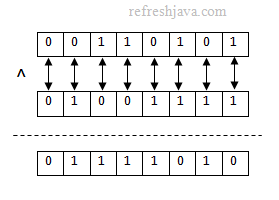### Bitwise complement operator

The unary bitwise complement operator `~` inverts the bit pattern of an operand. It converts `0` as `1` and `1` as `0`. For example a `byte` data type contains 8 bits, using this operator with a `byte` variable with value whose bit pattern is "`00110101`" would be changed as "`11001010`".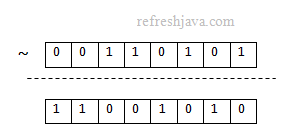### Bitwise operator program in Java

```` class` BitwiseOperatorDemo {
`public static void` main(String[] args) {
`byte` num1 = 53;
`byte` num2 = 79;
`int` result;

result = num1 & num2;
System.out.println(`"num1 & num2 = "` + result);

result = num1 | num2;
System.out.println(`"num1 | num2 = "` + result);

result = num1 ^ num2;
System.out.println(`"num1 ^ num2 = "` + result);

result = ~num1;  `/* 11001010(-54, 2's complement form) = ~00110101 */`
System.out.println(`"~num1 = "` + result );
}
}					```

Output:

num1 & num2 = 5
num1 | num2 = 127
num1 ^ num2 = 122
~num1 = -54

## Bit Shift operator in Java

As the name itself suggests, the bit shift operators shifts the bits of an operand to left or right depending on the shift operator used. In bit shift operator, the bit pattern(operand) is given in left-side while the number of positions to shift by is given in right side of the operator. All bit shift operators are binary operators in java as they requires two operands to operate. Table below shows the list of all bit shift operators in java.

Operator Name Example Description
<< Signed left shift Operator a << 2 Left shifts the bits of `a` by 2 position
>> Signed right shift Operator a >> 3 Right shifts the bits of `a` by 3 position.
>>> Unsigned right shift Operator a >>> 2 Right shifts the bits of `a` by 2 position and places 0's in left side.

### Signed left shift operator in Java

The signed left shift operator "`<<`" shifts a bit pattern to the left by the number of positions given in the right side of this operator. It places `0` in right side for every shift. Java stores number's in 2's complement notation(Refer wiki for more), in which most significant bit(leftmost bit) is used for sign bit. In java, if sign bit is `0`, it means the number is positive and if it is `1`, it means the number is negative.

Using this operator a negative number can become a positive number and vice-versa. If a `0` comes into sign bit after shifting the bits of a negative number, then it becomes a positive number. Similarly if `1` comes into sign bit after shifting the bits of a positive number, then it becomes a negative number. These conditions are indications of underflow and overflow respectively.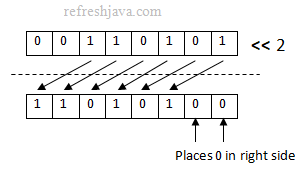### Signed right shift operator in java

The signed right shift operator "`>>`" shifts a bit pattern to the right by the number of positions given in the right side of this operator. The signed right shift operator places `0`(for +ve number) or `1`(for -ve number) in left side for every shift. That is why using this operator with a positive number will always be positive while a negative number will always be negative.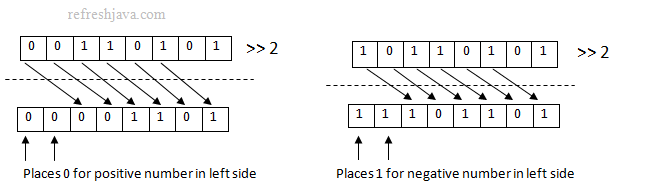### Unsigned right shift operator in java

The unsigned right shift operator "`>>>`" shifts a bit pattern to the right by the number of positions given in the right side of this operator and places a `0` into the leftmost position in every shift, no matter whether the number is negative or positive. That is why this operator always returns a positive number.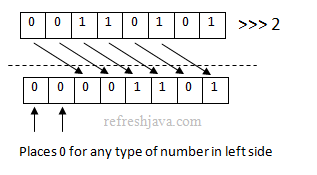### What is the difference between signed and unsigned right shift operator ?

The only difference between signed and unsigned right shift operator is, signed right shift operator places `0` or `1` into the leftmost position depending on whether the number is positive or negative while the unsigned right shift operator always places `0`, no matter the number is positive or negative. The result of >>> operator is always a positive number while result of >> operator is positive for positive numbers and negative for negative numbers.

### Bit Shift operators program in Java

```` class` BitShiftOperatorDemo {
`public static void` main(String[] args) {
`byte` num1 = 53;
`byte` num2 = -75;
`byte` result;

result = (`byte`)(num1 << 1);  `/* 01101010 = 00110101<<2 */`
System.out.println(`"num1 << 1 = "` + result);

result = (`byte`)(num1 << 2);  `/* 11010100 = 00110101<<2 */`
System.out.println(`"num1 << 2 = "` + result);

result = (`byte`)(num1 >> 2);  `/* 00001101 = 00110101>>2 */`
System.out.println(`"num1 >> 2  = "` + result);

result = (`byte`)(num1 >>> 2); `/* 00001101 = 00110101>>>2 */`
System.out.println(`"num1 >>> 2 = "` + result);
}
}					```

Note : By default operations in java returns an `int` type, that is why an explicit conversion has been done in above program.

Output:

num1 << 1 = 106
num1 << 2 = -44
num1 >> 2 = 13
num1 >>> 2 = 13

★★★
• Bitwise AND operation doesn't mean multiplication of two number.
• Bitwise OR operation doesn't mean addition of two number.
• The signed left shift << operator increases the value by 2 times for every shift if it doesn't fall's in underflow or overflow condition.
• For positive numbers, the the result of >> and >>> operators will be same.